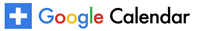The folded concave Laplacian spectral penalty learns block diagonal sparsity patterns with the strong oracle property
Online in Zoom
Online in Zoom

Structured sparsity is an important part of the modern statistical toolkit. We say a set of model parameters has block diagonal sparsity up to permutations if its elements can be viewed as the edges of a graph that has multiple connected components. For example, a block diagonal correlation matrix with K blocks of variables corresponds to a graph with K connected components whose nodes are the variables and whose edges are the correlations. This type of sparsity captures clusters of model parameters. To learn block diagonal sparsity patterns we develop folded concave Laplacian spectral penalty and provide a majorization-minimization algorithm for the resulting non-convex problem. We show this algorithm has the appealing computational and statistical guarantee of converging to the oracle estimator after two steps with high probability, even in high-dimensional settings. The theory is then demonstrated in several classical problems including covariance estimation, linear regression, and logistic regression.

# The folded concave Laplacian spectral penalty learns block diagonal sparsity patterns with the strong oracle property

### Biostatistics Seminar with Iain Carmichael, PhD: Postdoctoral Fellow Department of Pathology, Harvard Medical School and Memorial Sloan Kettering Cancer CenterJanuary 20, 2022
3:30 pm - 4:30 pm
Online in Zoom
Contact Information: Sabrina Olsson, siclayto@umich.edu

Structured sparsity is an important part of the modern statistical toolkit. We say a set of model parameters has block diagonal sparsity up to permutations if its elements can be viewed as the edges of a graph that has multiple connected components. For example, a block diagonal correlation matrix with K blocks of variables corresponds to a graph with K connected components whose nodes are the variables and whose edges are the correlations. This type of sparsity captures clusters of model parameters. To learn block diagonal sparsity patterns we develop folded concave Laplacian spectral penalty and provide a majorization-minimization algorithm for the resulting non-convex problem. We show this algorithm has the appealing computational and statistical guarantee of converging to the oracle estimator after two steps with high probability, even in high-dimensional settings. The theory is then demonstrated in several classical problems including covariance estimation, linear regression, and logistic regression.